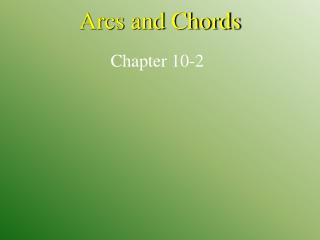DownloadDownload PresentationArcs and Chords

# Arcs and Chords

Télécharger la présentation## Arcs and Chords

- - - - - - - - - - - - - - - - - - - - - - - - - - - E N D - - - - - - - - - - - - - - - - - - - - - - - - - - -
##### Presentation Transcript

1. Arcs and Chords Chapter 10-2

2. Recognize major arcs, minor arcs, semicircles, and central angles and their measures. • Find arc length. • central angle • arc • minor arc • major arc • semicircle Standard 7.0Students prove and use theorems involving the properties of parallel lines cut by a transversal, the properties of quadrilaterals, and the properties of circles. (Key) Lesson 2 MI/Vocab

3. B C A Central Angle • The sum of the central angles of a circle = 360o • As long as they don’t overlap • An angle whose vertex is the center of the circle Central Angle BCA is a Central Angle

4. Lesson 2 Ex1

5. A • B • C • D A. 9 B. 21 C. 65 D. 30 Lesson 2 CYP1

6. A • B • C • D A. 15 B. 25 C. 40 D. 75 Lesson 2 CYP1

7. Arcs Def: A portion of a circle cut by two radii • Minor Arc—an arc formed by the interior of two radii with a central angle less than 180o • Major Arc—an arc formed by the exterior of two radii with a central angle less than 180o • Semi-Circle—an arc formed by the endpoints of a diameter • The measure of an arc is equal to the measure of the central angle that forms it • Two arcs are  if and only if their corresponding central angles are . (in the same circle or  circles) Animation: Arcs of a Circle

8. m AB = 60 ° m ADB = 300 ° Major Arc D B P ° 60 Minor Arc A

9. m AB = m DE = m AF = m DF = m BF = m BD = m DFB = m FE = Find the arc measures 80 ° 45 ° 45 ° 180 ° 55 ° 125 ° 45 ° 55 ° 305 ° 135 °

10. A m CA + m DC = 72 ° m CA = 40 ° m DA = 72 ° B C m DC = 32 ° D Arc Addition • The measure of an arc formed by two adjacent arcs is the sum of the measures of the 2 arcs

11. m CA + m DC = m AD = m ABD A m AC = 4x + 7 ° 8x ° B C m CD = 2x + 5 ° D Arc Addition Sample Problem • Find m ABD 4x + 7 + 2x + 5 = 8x 6x + 12 = 8x 12 = 2x 6 = x m ABD = 8(6) m ABD = 48 °

12. Measures of Arcs 46o Lesson 2 Ex2

13. is a minor arc, so is a semicircle. 46o Measures of Arcs Answer: 90 Lesson 2 Ex2

14. 46o Measures of Arcs Answer: 67 Lesson 2 Ex2

15. 46o Measures of Arcs 46o 44o Answer: 316 Lesson 2 Ex2

16. A • B • C • D A. 54 B. 27 C. 108 D. 72 Lesson 2 CYP2

17. A • B • C • D A. 54 B. 126 C. 108 D. 72 Lesson 2 CYP2

18. A • B • C • D A. 126 B. 234 C. 180 D. 288 Lesson 2 CYP2

19. Circle Graphs A. BICYCLES This graph shows the percent of each type of bicycle sold in the United States in 2001. Find the measurement of the central angle representing each category. Lesson 2 Ex3

20. Circle Graphs B. BICYCLES This graph shows the percent of each type of bicycle sold in the United States in 2001. Is the arc for the wedge named Youth congruent to the arc for the combined wedges named Other and Comfort? Answer: no Lesson 2 Ex3

21. Arc Length • Arc length is a part of the circumference of a circle. OR

22. A r = 12 cm B Find the Arc Length of AB mAB = 60o

23. In and . Write a proportion to compare each part to its whole. Arc Length Lesson 2 Ex4

24. degree measure of arc arc length circumference degree measure of whole circle Now solve the proportion for . Multiply each side by 9 . Answer: The length of is πunits or about 3.14 units. Arc Length Simplify. Lesson 2 Ex4

25. A • B • C • D A. 7.88 B. 15.75 C. 49.48 D. 24.74 Lesson 2 CYP4

26. Homework Chapter 10.2 • Pg 56711 - 28, 33 - 35 all